# Difference between revisions of "0708-1300/Class notes for Tuesday, April 1"

Announcements go here

## Typed Notes

The notes below are by the students and for the students. Hopefully they are useful, but they come with no guarantee of any kind.

### First Hour

Theorem

If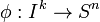$\phi:I^k\rightarrow S^n$ is an embbeding then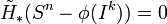$\tilde{H}_*(S^n-\phi(I^k)) = 0$

Proof:

By induction on k.

For k=0 this is easy,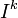$I^k$ is a single point and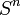$S^n$ - a point is just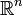$\mathbb{R}^n$

Now suppose we know the theorem is true for k-1, let$\phi:I^k\rightarrow S^n$ be an embedding. Write the cube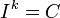$I^k = C$ as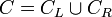$C=C_L\cup C_R$ where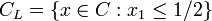$C_L=\{x\in C:x_1\leq 1/2\}$ and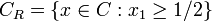$C_R=\{x\in C:x_1\geq 1/2\}$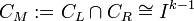$C_M:= C_L\cap C_R\cong I^{k-1}$

So,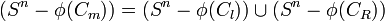$(S^n-\phi(C_m)) = (S^n-\phi(C_l))\cup(S^n-\phi(C_R))$

where both sets in the union are open. Recall for sets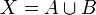$X = A\cup B$ where A and B are open we have the Mayer Vietoris Sequence.

Note: It is usefull to "open the black box" and think about the Mayer Vietoris Sequence from the veiwpoint of singular homology, ie using maps of simplexes and the like. This was briefly sketched in class.

We get a sequence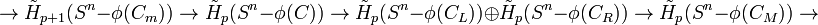$\rightarrow\tilde{H}_{p+1}(S^n-\phi(C_m))\rightarrow \tilde{H}_{p}(S^n-\phi(C))\rightarrow \tilde{H}_{p}(S^n-\phi(C_L))\oplus \tilde{H}_{p}(S^n-\phi(C_R))\rightarrow \tilde{H}_{p}(S^n-\phi(C_M))\rightarrow$

The first and last terms in this sequence (at least the small part of it we have written) vanish by induction hypothesis.

Note: Technically we need to check at Mayer Vietoris sequence also works for reduced homology.

We thus get the isomorphism: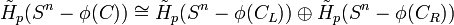$\tilde{H}_{p}(S^n-\phi(C))\cong \tilde{H}_{p}(S^n-\phi(C_L))\oplus \tilde{H}_{p}(S^n-\phi(C_R))$

Assume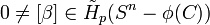$0\neq[\beta]\in \tilde{H}_{p}(S^n-\phi(C))$ As the above isormorphism is induced by the inclusion maps at the chain level, we get that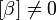$[\beta]\neq 0$ either in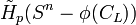$\tilde{H}_{p}(S^n-\phi(C_L))$ or in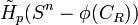$\tilde{H}_{p}(S^n-\phi(C_R))$

Now repeat, find a sequence of intervals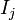$I_j$ such that

1)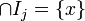$\cap I_j = \{x\}$

2)$[\beta]\neq 0$ in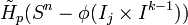$\tilde{H}_{p}(S^n-\phi(I_j\times I^{k-1}))$

Pictorially, we cut C in half, find which half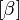$[\beta]$ is non zero, then cut this half in half and find the one the$[\beta]$ is non zero in and again cut it in half, etc...

But,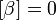$[\beta] = 0$ in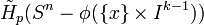$\tilde{H}_{p}(S^n-\phi(\{x\}\times I^{k-1}))$ by induction. Hence,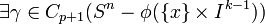$\exists\gamma\in C_{p+1}(S^n-\phi(\{x\}\times I^{k-1}))$ such that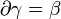$\partial\gamma=\beta$.

But,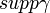$supp\gamma$ = { union of images of simplexes in U} is compact, so,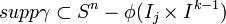$supp\gamma\subset S^n-\phi(I_j\times I^{k-1})$ for some j. But this contradicts assumption 2)

Q.E.D

We now prove an analogous theorem for spheres opposed to cubes

Theorem

For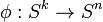$\phi:S^k\rightarrow S^n$ an embedding then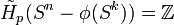$\tilde{H}_{p}(S^n-\phi(S^k)) = \mathbb{Z}$ if p = n-k-1 and zero otherwise.

Intuitively,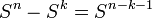$S^n-S^k = S^{n-k-1}$

Recall we had seen this explicitly for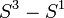$S^3-S^1$ when we wrote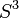$S^3$ as the union of two tori (an inflated circle)

Proof:

As in the previous proof, we use Mayer Vietoris. Let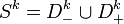$S^k = D^k_-\cup D^k_+$

Then,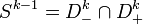$S^{k-1} = D^k_-\cap D^k_+$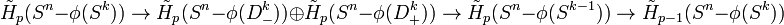$\tilde{H}_{p}(S^n-\phi(S^k))\rightarrow \tilde{H}_{p}(S^n-\phi(D^k_-))\oplus\tilde{H}_{p}(S^n-\phi(D^k_+))\rightarrow \tilde{H}_{p}(S^n-\phi(S^{k-1}))\rightarrow \tilde{H}_{p-1}(S^n-\phi(S^k))$

The first and last term written vanish by induction hypothesis and so have an isomorphism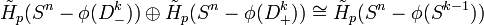$\tilde{H}_{p}(S^n-\phi(D^k_-))\oplus\tilde{H}_{p}(S^n-\phi(D^k_+))\cong \tilde{H}_{p}(S^n-\phi(S^{k-1}))$

Hence, it is enough to prove the theorem for k=0. Well in this case we have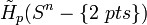$\tilde{H}_{p}(S^n-\{2\ pts\})$

Since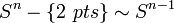$S^n-\{2\ pts\}\sim S^{n-1}$, this is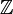$\mathbb{Z}$ for p = n-1 and 0 otherwise

Q.E.D

Take k=n-1$\tilde{H}_{0}(S^n-\phi(S^{n-1})) =\mathbb{Z}$ so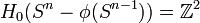$H_{0}(S^n-\phi(S^{n-1}))=\mathbb{Z}^2$

Hence the compliment of an n-1 sphere in$S^n$ has two connected components. This is the Jordan Curve Theorem.

### Second Hour

Excersize

Consider the embedding of a line in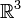$\mathbb{R}^3$ which wraps arround somewhat like a helix only each loop makes a link with each previous loop and in addition the helical object gets smaller and converges to two points at the end.

The question is, should we put a little circle L about a part of the helical object can we make this L the boundary of a disk embedded in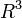$R^3$.

Sketch of Answer: Consider the bubble which has L as the boundary and goes all the way arround the object at one end. This is not an embedding since there are two intersection points with the helical object. Cut two small holes from the bubble at each of these points. This is still not quite it since the boundary also has these two small holes. Hence connect the two holes via a tubular neighbourhood going along the helical object and we have the result.

The real excersize here is to figure out how the Mayer Vietoris sequence actually determines this particular surface.

Proposition

An open path connected set in$\mathbb{R}^n$ is clopen connected.

Proof This is easy, just using general topology

Hence, for open sets in$\mathbb{R}^n$ path components are the same as clopen components

Theorem

"Invariance of Domain" or "Openness in$\mathbb{R}^n$ is intrinsic"

If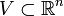$V\subset\mathbb{R}^n$ is homeomorphic to an open set in$\mathbb{R}^n$ then it is an open set in$\mathbb{R}^n$

Here are two examples that illustrate why this might not be obvious

1) Replacing open with dense would not be true, for instance, the rationals in [0,1] are not dence in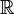$\mathbb{R}$ but they ARE homeomorphic to the rationals which ARE dense in R.

2) Replacing open with closed is also not true, for instance,$\mathbb{R}^n$ is closed in$\mathbb{R}^n$

but it is also homeomorphic to the open ball which is not closed.

Proof

Let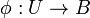$\phi:U\rightarrow B$

Consider a ball B in U, with boundary the circle S and we get a disk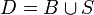$D=B\cup S$

We have that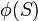$\phi(S)$ devides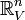$\mathbb{R}^n_V$ into two connected components. Call them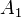$A_1$ and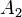$A_2$.

We have both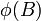$\phi(B)$ and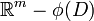$\mathbb{R}^m - \phi(D)$ but we need to show these ARE the two connected components. We first note that both are in fact connected.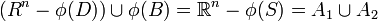$(R^n -\phi(D))\cup\phi(B) = \mathbb{R}^n-\phi(S) = A_1\cup A_2$

Hence$\phi(B)$ is one of the components and so it is open. Q.E.D

Theorem Borsuk-Ulam

If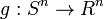$g:S^n\rightarrow R^n$ is continuous then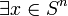$\exists x\in S^n$ such that g(x) = g(-x)

Corrollary The Ham Sandwich Theorem (or Salad Bowl Theorem, according to Dr. Bar Natan)

Loosely this says that if we have n objects in a bounded domain of$\mathbb{R}^n$ such as items in a salad or sandwhich then we can find an n-1 dimensional hyperplane such that the hyperplane precisely cuts each item in half.

Formally, If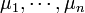$\mu_1,\cdots,\mu_n$ are densities in$\mathbb{R}^n$ with bounded support then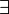$\exists$ a hyperplane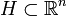$H\subset\mathbb{R}^n$ deviding$\mathbb{R}^n$ into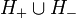$H_+\cup H_-$ such that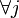$\forall j$,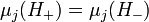$\mu_j(H_+) = \mu_j(H_-)$

Proof:

Consider the set of sided hyperplanes in$\mathbb{R}^n$ which equals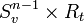$S^{n-1}_v\times R_t$ where the first component determines the normal vector v and the second component determines how far away this plane is front the origin in the direction of v.

Define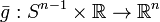$\bar{g}:S^{n-1}\times\mathbb{R}\rightarrow\mathbb{R}^n$ via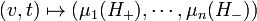$(v,t)\mapsto (\mu_1(H_+),\cdots, \mu_n(H_-))$

Now, if t>>0, clearly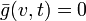$\bar{g}(v,t) = 0$ and if t<<0 then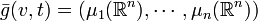$\bar{g}(v,t) = (\mu_1(\mathbb{R}^n),\cdots,\mu_n(\mathbb{R}^n))$

Hence we have a cylinder mapping into$\mathbb{R}^n$ such that it is constant along the top and bottom of the cylindar. Hence, we can pinch the top and bottom of the cylinder. We thus get a map g from$S^n$ to$\mathbb{R}^n$ and, applying Borsuk-Ulam, deduce there is a point such that g(x) = g(-x) and so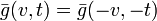$\bar{g}(v,t) = \bar{g}(-v,-t)$ for some point (v,t). Hence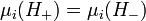$\mu_i(H_+)=\mu_i(H_-)$ for all i

Q.E.D